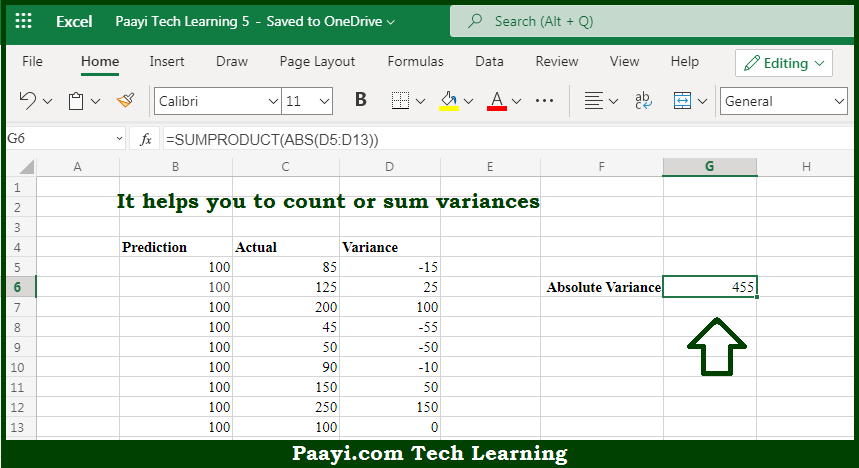# Learn How to Count or Sum Variance in Microsoft Excel

Written by | 0 Comments | 501 Views

In this article, you will learn how to COUNT various things in Microsoft Excel using a single or combination of functions and its purpose in Microsoft Excel. You will also get to know how to Count or Sum Variance and see the generic formula.

Count or Sum Variance in Microsoft Excel

The main purpose of this formula is to count or sum variances. Here we will learn how to count either count or sum variance in Microsoft Excel. That implies, with the help of a formula based on the SUMPRODUCT and ABS functions you can able to count or sum variances. So, with the help of this formula, you can able to how to count either count or sum variance

General Formula to Count or Sum Variance

=SUMPRODUCT(ABS(variance))

The Explanation for the Count or Sum VarianceSo we know that with the help of the given formula above you can able to count or sum variances. Here we will learn how to count either count or sum variance in Microsoft Excel. As we know that, the main purpose of the example provided here is to sum or count a set of variances in various ways. As you can see that we have listed the variances in D5:D15, which is also the named range variance. It should be noted that the first formula in F5 is only used to sum all variances with the SUM function. So, with the help of this formula, you can able to count r sum variances.